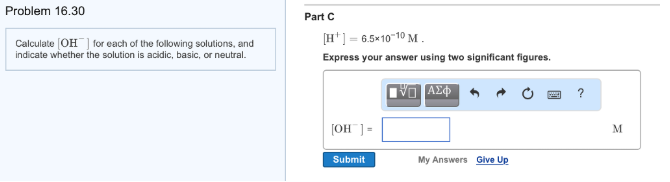# Problem: Calculate [OH-] for each of the following solutions, and indicate whether the solution is acidic, basic, or neutral. [H+] = 6.5 x 10-10 M. Express your answer using two significant figures.

###### FREE Expert Solution
97% (185 ratings)###### Problem Details

Calculate [OH-] for each of the following solutions, and indicate whether the solution is acidic, basic, or neutral.

[H+] = 6.5 x 10-10 M. Express your answer using two significant figures.